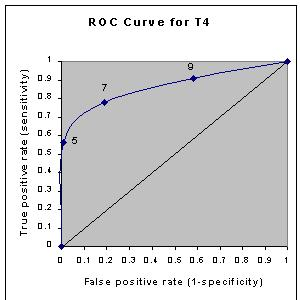## Plotting and Intrepretating an ROC Curve

| Previous Section | Main Menu | Next Section |
This section continues the hypothyroidism example started in the the previous section. We showed that the table at left can be summarized by the operating characteristics at right:

 T4 value Hypothyroid Euthyroid 5 or less 18 1 5.1 - 7 7 17 7.1 - 9 4 36 9 or more 3 39 Totals: 32 93
 Cutpoint Sensitivity Specificity 5 0.56 0.99 7 0.78 0.81 9 0.91 0.42

The operating characteristics (above right) can be reformulated slightly and then presented graphically as shown below to the right:

 Cutpoint True Positives False Positives 5 0.56 0.01 7 0.78 0.19 9 0.91 0.58This type of graph is called a Receiver Operating Characteristic curve (or ROC curve.) It is a plot of the true positive rate against the false positive rate for the different possible cutpoints of a diagnostic test.

An ROC curve demonstrates several things:

1. It shows the tradeoff between sensitivity and specificity (any increase in sensitivity will be accompanied by a decrease in specificity).
2. The closer the curve follows the left-hand border and then the top border of the ROC space, the more accurate the test.
3. The closer the curve comes to the 45-degree diagonal of the ROC space, the less accurate the test.
4. The slope of the tangent line at a cutpoint gives the likelihood ratio (LR) for that value of the test. You can check this out on the graph above. Recall that the LR for T4 < 5 is 52. This corresponds to the far left, steep portion of the curve. The LR for T4 > 9 is 0.2. This corresponds to the far right, nearly horizontal portion of the curve.
5. The area under the curve is a measure of text accuracy. This is discussed further in the next section.

.
| Previous Section | Main Menu | Next Section |